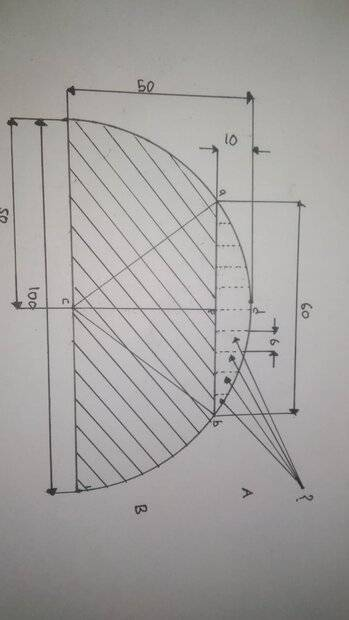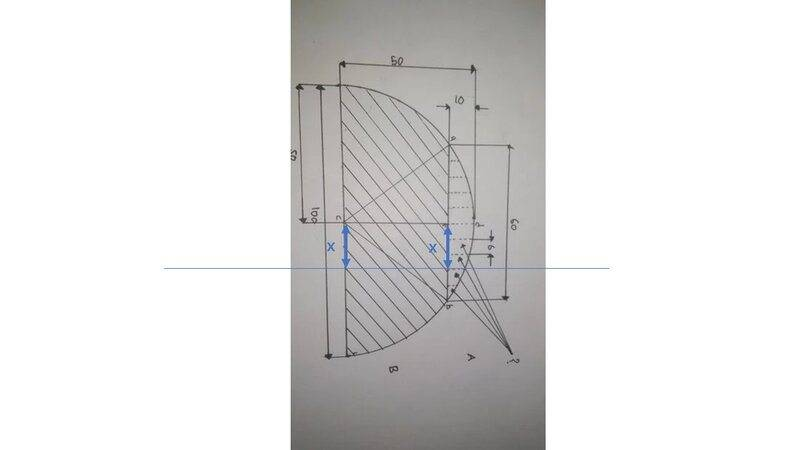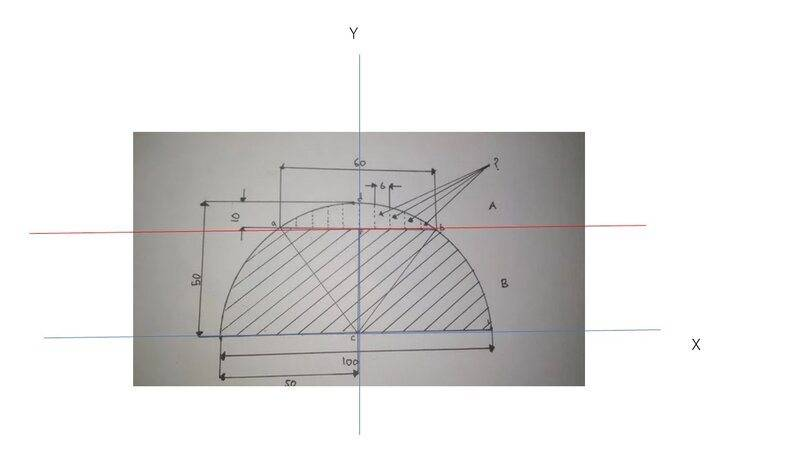# Easy geometry problem, please give me a hint...

Homework Statement:
I'm struggling to find an equation to find the distance between two points from the segment to the arc of the circle.
Relevant Equations:
I'm thinking of just using Pythagorean Theorem, but it doesn't work for the segment of a circle. Circle equations.I have calculated the height of the segment using the Pythagorean Theorem and that's currently where I am right now. I don't seem to find any equations that can help me. Though I might be not trying hard enough or using the wrong words because I'm not really fluent in mathematical terms as you can observe from the details that I provided.

EDIT: I might be in the wrong topics, but whatever.

anuttarasammyak
Gold Member
$$\sqrt{50^2-x^2}-10$$where x is distance from c line.

$$\sqrt{50^2-x^2}-10$$where x is distance from c line.
Kindly explain where the equation come from?

The equation of circle ref. https://en.m.wikipedia.org/wiki/Circle
$$\sqrt{50^2-x^2}-10$$where x is distance from c line.
What did you mean by the "distance from c line" sorry? From what point?

Last edited by a moderator:
anuttarasammyak
Gold Member
Edit. Not -10 but -40. Say x＝０how much is it and where is it ?

Mark44
Mentor
manareus said:
I'm struggling to find an equation to find the distance between two points from the segment to the arc of the circle.
It's not clear to me what you're trying to find. Which two points on the line segment?

Also, a segment of a circle is a portion of the circle subtended by two radii, sort of like a piece of pie.

•WWGD and Delta2
Edit. Not -10 but -40. Say x＝０how much is it and where is it ?
It should be 10 and it's the sagitta, am I correct?

But I'm still not sure how can you come up with that equation though. Yes it's the equation of a circle but, if I'm wording this correctly, where did you derived this equation from?

Is it from C = πd? Or A = πr^2?

Or even r^2 = x^2 + y^2? Please guide me through it. I don't like not knowing the aspect of "why" from an equation.

Last edited:
It's not clear to me what you're trying to find. Which two points on the line segment?

Also, a segment of a circle is a portion of the circle subtended by two radii, sort of like a piece of pie.
I'm trying to find the distance between the chord to the arc of the circle, see question mark I put on the picture I shared. Sorry if I make you confused.

PeroK
Homework Helper
Gold Member
2020 Award
I'm trying to find the distance between the chord to the arc of the circle, see question mark I put on the picture I shared. Sorry if I make you confused.
That distance varies depending on the point of the chord you choose. Are you trying to find the distance at points 6 units apart on the chord?

I would rotate your diagram anticlockwise and write down the equation of the chord and the circle. The distance is simply the difference in y-coordinates for a given x-coordinate.

•Steve4Physics
anuttarasammyak
Gold Member
It should be 10 and it's the sagitta, am I correct?
So you see x asanuttarasammyak
Gold Member
I would rotate your diagram anticlockwise and write down the equation of the chord and the circle. The distance is simply the difference in y-coordinates for a given x-coordinate.@manareus Do you write down equations of this upper half circle and the red horizontal line ?

•PeroK

That distance varies depending on the point of the chord you choose. Are you trying to find the distance at points 6 units apart on the chord?

I would rotate your diagram anticlockwise and write down the equation of the chord and the circle. The distance is simply the difference in y-coordinates for a given x-coordinate.
Interesting, though I cannot admit that I am able to visualize it.
View attachment 290568

@manareus Do you write down equations of this upper half circle and the red horizontal line ?
Hmm I don't know actually my Professor doesnt teach me any chord equations. Probably only 1, where if one bowstring is the diameter and the other bowstring cuts perpendicularly, it will cut into two equal lengths.

Regarding the equation for a circle you mean the area or the circumference? Or the x and y one?

anuttarasammyak
Gold Member
Or even r^2 = x^2 + y^2?
Yes, that's it.

Last edited:
PeroK
Homework Helper
Gold Member
2020 Award
Interesting, though I cannot admit that I am able to visualize it.
See post #12.

Hmm I don't know actually my Professor doesnt teach me any chord equations.
In post #12 the chord is a horizontal line. You shouldn't need a professor to tell you the equation of one of those!

Yes, that's it.
See post #12.

In post #12 the chord is a horizontal line. You shouldn't need a professor to tell you the equation of one of those!
Ah okay thank you for your help!

WWGD
Gold Member
Homework Statement:: I'm struggling to find an equation to find the distance between two points from the segment to the arc of the circle.
Relevant Equations:: I'm thinking of just using Pythagorean Theorem, but it doesn't work for the segment of a circle. Circle equations.

View attachment 290504
I have calculated the height of the segment using the Pythagorean Theorem and that's currently where I am right now. I don't seem to find any equations that can help me. Though I might be not trying hard enough or using the wrong words because I'm not really fluent in mathematical terms as you can observe from the details that I provided.

EDIT: I might be in the wrong topics, but whatever.
Could you please indicate just what segments you're referring to here? Maybe mark them in red or other color?

gmax137
•